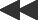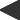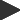basis: julia-fractal z(0) = pixel; z(n + 1) = z(n)² + c2 parameters: real and imaginary parts of c. These sets were named for mathematician Gaston Julia, and can be generated by a simple change in the iteration process described for the Mandelbrot Set. Start with a specified value of C, "C-real + i * C-imaginary"; use as the initial value of Z "x-coordinate + i * y-coordinate"; and repeat the same iteration,Z(n+1) = Z(n)² + C.The relationship between the Mandelbrot set and Julia set can hold between other sets as well. All these are generated by equations that are of the form z(k+1) = f[z(k),c], where the function orbit is the sequence z(0), z(1), ..., and the variable c is a complex parameter of the equation.The value c is fixed for "Julia" sets. The initial orbit value z(0) is the complex number corresponding to the screen pixel.